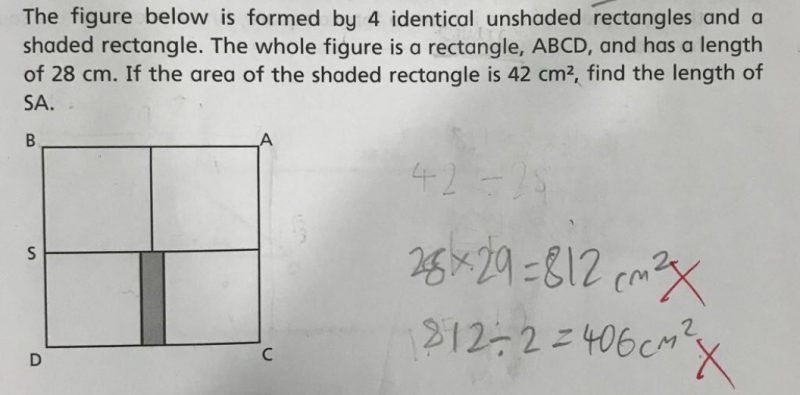# QuestionThis is a area question.

1. BA = 28. So length of rectangle is 14 cm.

2.  The figure is out of shape. SD = 14.

42 ÷ 14 = 3 cm .(shaded rectangle )

Breadth = (28 – 3) / 2  = 12.5

3. It could be typo error. So SB = 12.5 cm.

😊

0 Replies 0 Likes

DOn’t understand the question, when it asked for the length of SA? SA is the diagonal.

OR does SA just mean shaded area?

0 Replies 0 Likes